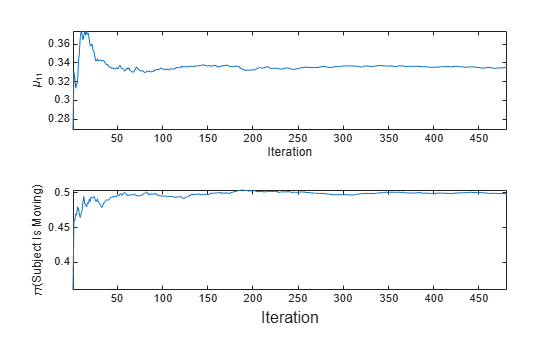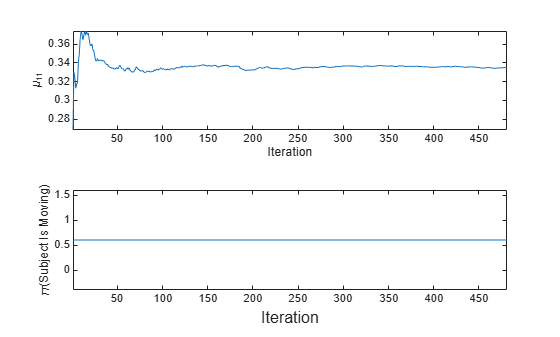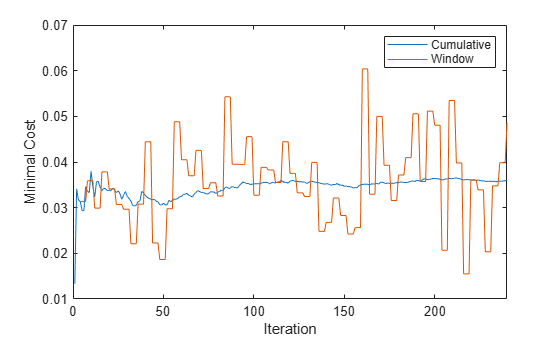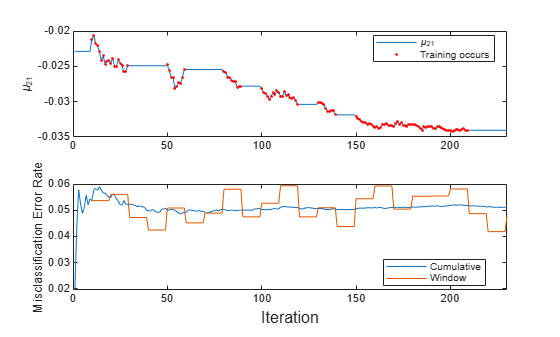Main Content

# fit

Train naive Bayes classification model for incremental learning

## Syntax

Mdl = fit(Mdl,X,Y)
Mdl = fit(Mdl,X,Y,'Weights',Weights)

## Description

The fit function fits a configured naive Bayes classification model for incremental learning (incrementalClassificationNaiveBayes object) to streaming data. To additionally track performance metrics using the data as it arrives, use updateMetricsAndFit instead.

To fit or cross-validate a naive Bayes classification model to an entire batch of data at once, see fitcnb.

example

Mdl = fit(Mdl,X,Y) returns a naive Bayes classification model for incremental learning Mdl, which represents the input naive Bayes classification model for incremental learning Mdl trained using the predictor and response data, X and Y respectively. Specifically, fit updates the conditional posterior distribution of the predictor variables given the data.

example

Mdl = fit(Mdl,X,Y,'Weights',Weights) specifies observation weights Weights.

## Examples

collapse all

This example shows how to fit an incremental naive Bayes learner when you know only the expected maximum number of classes in the data.

Create a incremental naive Bayes model. Specify that the maximum number of expected classes is 5.

Mdl = incrementalClassificationNaiveBayes('MaxNumClasses',5)
Mdl = incrementalClassificationNaiveBayes IsWarm: 0 Metrics: [1×2 table] ClassNames: [1×0 double] ScoreTransform: 'none' DistributionNames: 'normal' DistributionParameters: {} Properties, Methods 

Mdl is an incrementalClassificationNaiveBayes model. All its properties are read-only. Mdl can encounter at most 5 unique classes. By default, the prior class distribution Mdl.Prior is empirical, which means the software updates the prior distribution as it encounters labels.

Mdl must be fit to data before you can use it to perform any other operations.

Load the human activity data set. Randomly shuffle the data.

load humanactivity n = numel(actid); rng(1) % For reproducibility idx = randsample(n,n); X = feat(idx,:); Y = actid(idx);

For details on the data set, enter Description at the command line.

Fit the incremental model to the training data, in chunks of 50 observations at a time by using the fit function. At each iteration:

• Simulate a data stream by processing 50 observations.

• Overwrite the previous incremental model with a new one fitted to the incoming observation.

• Store the mean of the first predictor in the first class ${\mu }_{11}$ and the prior probability that the subject is moving (Y > 2) to see how they evolve during incremental training.

% Preallocation numObsPerChunk = 50; nchunk = floor(n/numObsPerChunk); mu11 = zeros(nchunk,1); priormoved = zeros(nchunk,1); % Incremental fitting for j = 1:nchunk ibegin = min(n,numObsPerChunk*(j-1) + 1); iend = min(n,numObsPerChunk*j); idx = ibegin:iend; Mdl = fit(Mdl,X(idx,:),Y(idx)); mu11(j) = Mdl.DistributionParameters{1,1}(1); priormoved(j) = sum(Mdl.Prior(Mdl.ClassNames > 2)); end

IncrementalMdl is an incrementalClassificationNaiveBayes model object trained on all the data in the stream.

To see how the parameters evolved during incremental learning, plot them on separate subplots.

figure; subplot(2,1,1) plot(mu11) ylabel('\mu_{11}') xlabel('Iteration') axis tight subplot(2,1,2) plot(priormoved); ylabel('Prior P(Subject Moved)') xlabel('Iteration') axis tightfit updates the posterior mean of the predictor distribution as it processes each chunk. Because the prior class distribution is empirical, $\pi$(subject is moving) changes as fit processes each chunk.

This example shows how to fit an incremental naive Bayes learner when you know all the class names in the data.

Consider training a device to predict whether a subject is sitting, standing, walking, running, or dancing based on biometric data measured on the subject, and you know the class names map 1 through 5 to an activity. Also, suppose that the researchers plan to expose the device to each class uniformly.

Create an incremental naive Bayes learner for multiclass learning. Specify the class names and a uniform prior class distribution.

classnames = 1:5; Mdl = incrementalClassificationNaiveBayes('ClassNames',classnames,'Prior','uniform')
Mdl = incrementalClassificationNaiveBayes IsWarm: 0 Metrics: [1×2 table] ClassNames: [1 2 3 4 5] ScoreTransform: 'none' DistributionNames: 'normal' DistributionParameters: {5×0 cell} Properties, Methods 

Mdl is an incrementalClassificationNaiveBayes model object. All its properties are read-only. During training, observed labels must be in Mdl.ClassNames.

Mdl must be fit to data before you can use it to perform any other operations.

Load the human activity data set. Randomly shuffle the data.

load humanactivity n = numel(actid); rng(1); % For reproducibility idx = randsample(n,n); X = feat(idx,:); Y = actid(idx);

For details on the data set, enter Description at the command line.

Fit the incremental model to the training data by using the fit function. Simulate a data stream by processing chunks of 50 observations at a time. At each iteration:

• Process 50 observations.

• Overwrite the previous incremental model with a new one fitted to the incoming observation.

• Store the mean of the first predictor in the first class ${\mu }_{11}$ and the prior probability that the subject is moving (Y > 2) to see how they evolve during incremental training.

% Preallocation numObsPerChunk = 50; nchunk = floor(n/numObsPerChunk); mu11 = zeros(nchunk,1); priormoved = zeros(nchunk,1); % Incremental fitting for j = 1:nchunk ibegin = min(n,numObsPerChunk*(j-1) + 1); iend = min(n,numObsPerChunk*j); idx = ibegin:iend; Mdl = fit(Mdl,X(idx,:),Y(idx)); mu11(j) = Mdl.DistributionParameters{1,1}(1); priormoved(j) = sum(Mdl.Prior(Mdl.ClassNames > 2)); end

IncrementalMdl is an incrementalClassificationNaiveBayes model object trained on all the data in the stream.

To see how the parameters evolved during incremental learning, plot them on separate subplots.

figure; subplot(2,1,1) plot(mu11) ylabel('\mu_{11}') xlabel('Iteration') axis tight subplot(2,1,2) plot(priormoved); ylabel('Prior P(Subject Moved)') xlabel('Iteration') axis tightfit updates the posterior mean of the predictor distribution as it processes each chunk. Because the prior class distribution is specified as uniform, $\pi$(subject is moving) = 0.6 and does not change as fit processes each chunk.

Train a naive Bayes classification model by using fitcnb, convert it to an incremental learner, track its performance on streaming data, and then fit it to the data. Specify observation weights.

Load and Preprocess Data

Load the human activity data set. Randomly shuffle the data.

load humanactivity rng(1); % For reproducibility n = numel(actid); idx = randsample(n,n); X = feat(idx,:); Y = actid(idx);

For details on the data set, enter Description at the command line.

Suppose that the data collected when the subject was not moving (Y <= 2) has double the quality than when the subject was moving. Create a weight variable that attributes 2 to observations collected from a still subject, and 1 to a moving subject.

W = ones(n,1) + ~Y;

Train Naive Bayes Classification Model

Fit a naive Bayes classification model to a random sample of half the data.

idxtt = randsample([true false],n,true); TTMdl = fitcnb(X(idxtt,:),Y(idxtt),'Weights',W(idxtt))
TTMdl = ClassificationNaiveBayes ResponseName: 'Y' CategoricalPredictors: [] ClassNames: [1 2 3 4 5] ScoreTransform: 'none' NumObservations: 12053 DistributionNames: {1×60 cell} DistributionParameters: {5×60 cell} Properties, Methods 

TTMdl is a ClassificationNaiveBayes model object representing a traditionally trained naive Bayes classification model.

Convert Trained Model

Convert the traditionally trained model to a naive Bayes classification for incremental learning.

IncrementalMdl = incrementalLearner(TTMdl)
IncrementalMdl = incrementalClassificationNaiveBayes IsWarm: 1 Metrics: [1×2 table] ClassNames: [1 2 3 4 5] ScoreTransform: 'none' DistributionNames: {1×60 cell} DistributionParameters: {5×60 cell} Properties, Methods 

IncrementalMdl is an incrementalClassificationNaiveBayes model. Because class names are specified in Mdl.ClassNames, labels encountered during incremental learning must be in Mdl.ClassNames.

Separately Track Performance Metrics and Fit Model

Perform incremental learning on the rest of the data by using the updateMetrics and fit functions. At each iteration:

1. Simulate a data stream by processing 50 observations at a time.

2. Call updateMetrics to update the cumulative and window classification error of the model given the incoming chunk of observations. Overwrite the previous incremental model to update the losses in the Metrics property. Note that the function does not fit the model to the chunk of data—the chunk is "new" data for the model. Specify the observation weights.

3. Call fit to fit the model to the incoming chunk of observations. Overwrite the previous incremental model to update the model parameters. Specify the observation weights.

4. Store the minimal cost.

% Preallocation idxil = ~idxtt; nil = sum(idxil); numObsPerChunk = 50; nchunk = floor(nil/numObsPerChunk); mc = array2table(zeros(nchunk,2),'VariableNames',["Cumulative" "Window"]); Xil = X(idxil,:); Yil = Y(idxil); Wil = W(idxil); % Incremental fitting for j = 1:nchunk ibegin = min(nil,numObsPerChunk*(j-1) + 1); iend = min(nil,numObsPerChunk*j); idx = ibegin:iend; IncrementalMdl = updateMetrics(IncrementalMdl,Xil(idx,:),Yil(idx),... 'Weights',Wil(idx)); mc{j,:} = IncrementalMdl.Metrics{"MinimalCost",:}; IncrementalMdl = fit(IncrementalMdl,Xil(idx,:),Yil(idx),'Weights',Wil(idx)); end

IncrementalMdl is an incrementalClassificationNaiveBayes model object trained on all the data in the stream.

Alternatively, you can use updateMetricsAndFit to update performance metrics of the model given a new chunk of data, and then fit the model to the data.

Plot a trace plot of the performance metrics.

h = plot(mc.Variables); xlim([0 nchunk]); ylabel('Minimal Cost') legend(h,mc.Properties.VariableNames) xlabel('Iteration')The cumulative loss gradually stabilizes, whereas the window loss jumps.

Incrementally train a naive Bayes classification model only when its performance degrades.

Load the human activity data set. Randomly shuffle the data.

load humanactivity n = numel(actid); rng(1) % For reproducibility idx = randsample(n,n); X = feat(idx,:); Y = actid(idx);

For details on the data set, enter Description at the command line.

Configure a naive Bayes classification model for incremental learning so that the maximum number of expected classes is 5, the tracked performance metric includes the misclassification error rate, and the metrics window size of 1000. Fit the configured model to the first 1000 observations.

Mdl = incrementalClassificationNaiveBayes('MaxNumClasses',5,'MetricsWindowSize',1000,... 'Metrics','classiferror'); initobs = 1000; Mdl = fit(Mdl,X(1:initobs,:),Y(1:initobs));

Mdl is an incrementalClassificationNaiveBayes model object.

Perform incremental learning, with conditional fitting, by following this procedure for each iteration:

• Simulate a data stream by processing a chunk of 100 observations at a time.

• Update the model performance on the incoming chunk of data.

• Fit the model to the chunk of data only when the misclassification error rate is greater than 0.05.

• When tracking performance and fitting, overwrite the previous incremental model.

• Store the misclassification error rate and the mean of the first predictor in the second class ${\mu }_{21}$ to see how they evolve during training.

• Track when fit trains the model.

% Preallocation numObsPerChunk = 100; nchunk = floor((n - initobs)/numObsPerChunk); mu21 = zeros(nchunk,1); ce = array2table(nan(nchunk,2),'VariableNames',["Cumulative" "Window"]); trained = false(nchunk,1); % Incremental fitting for j = 1:nchunk ibegin = min(n,numObsPerChunk*(j-1) + 1 + initobs); iend = min(n,numObsPerChunk*j + initobs); idx = ibegin:iend; Mdl = updateMetrics(Mdl,X(idx,:),Y(idx)); ce{j,:} = Mdl.Metrics{"ClassificationError",:}; if ce{j,2} > 0.05 Mdl = fit(Mdl,X(idx,:),Y(idx)); trained(j) = true; end mu21(j) = Mdl.DistributionParameters{2,1}(1); end

Mdl is an incrementalClassificationNaiveBayes model object trained on all the data in the stream.

To see how the model performance and ${\mu }_{21}$ evolved during training, plot them on separate subplots.

subplot(2,1,1) plot(mu21) hold on plot(find(trained),mu21(trained),'r.') ylabel('\mu_{21}') legend('\mu_{21}','Training occurs','Location','best') hold off subplot(2,1,2) plot(ce.Variables) ylabel('Misclassification Error Rate') xlabel('Iteration') legend(ce.Properties.VariableNames,'Location','best')The trace plot of ${\mu }_{21}$ shows periods of constant values, during which the loss within the previous 1000 observation window is at most 0.05.

## Input Arguments

collapse all

Naive Bayes classification model for incremental learning to fit to streaming data, specified as an incrementalClassificationNaiveBayes model object. You can create Mdl directly or by converting a supported, traditionally trained machine learning model using the incrementalLearner function. For more details, see the corresponding reference page.

Chunk of predictor data to which the model is fit, specified as an n-by-Mdl.NumPredictors floating point matrix.

The length of the observation labels Y and the number of observations in X must be equal; Y(j) is the label of observation j (row or column) in X.

Note

• If Mdl.NumPredictors = 0, fit infers the number of predictors from X, and sets the congruent property of the output model. Otherwise, if the number of predictor variables in the streaming data changes from Mdl.NumPredictors, fit issues an error.

• fit supports only floating-point input predictor data. If the input model Mdl represents a converted, traditionally trained model fit to categorical data, use dummyvar to convert each categorical variable to a numeric matrix of dummy variables, and concatenate all dummy variable matrices and any other numeric predictors. For more details, see Dummy Variables.

Data Types: single | double

Chunk of labels to which the model is fit, specified as a categorical, character, or string array, logical or floating-point vector, or cell array of character vectors.

The length of the observation labels Y and the number of observations in X must be equal; Y(j) is the label of observation j (row or column) in X. fit issues an error when at least one of the conditions is met:

• Y contains a newly encountered label and the maximum number of classes has been reached previously (see MaxNumClasses and ClassNames arguments of incrementalClassificationNaiveBayes).

• The data types of Y and Mdl.ClassNames are different.

Data Types: char | string | cell | categorical | logical | single | double

Chunk of observation weights, specified as a floating-point vector of positive values. fit weighs the observations in X with the corresponding values in Weights. The size of Weights must equal n, which is the number of observations in X.

By default, Weights is ones(n,1).

For more details, including normalization schemes, see Observation Weights.

Data Types: double | single

Note

If an observation (predictor or label) or weight contains at least one missing (NaN) value, fit ignores the observation. Consequently, fit uses fewer than n observations to compute the model performance.

## Output Arguments

collapse all

Updated naive Bayes classification model for incremental learning, returned as an incremental learning model object of the same data type as the input model Mdl, an incrementalClassificationNaiveBayes object.

If the ClassNames property of the input model Mdl is an empty array, fit sets the ClassNames property of the output model Mdl to unique(Y). If the maximum number of classes is not reached, fit appends to Mdl.ClassNames any newly encountered labels in Y.

## Tips

• Unlike traditional training, incremental learning might not have a separate test (holdout) set. Therefore, to treat each incoming chunk of data as a test set, pass the incremental model and each incoming chunk to updateMetrics before training the model on the same data.

## Algorithms

collapse all

### Observation Weights

For each conditional predictor distribution, fit computes the weighted average and standard deviation.

If the prior class probability distribution is known (in other words, the prior distribution is not empirical), fit normalizes observation weights to sum to the prior class probabilities in the respective classes. This action implies that the default observation weights are the respective prior class probabilities.

If the prior class probability distribution is empirical, the software normalizes the specified observation weights to sum to 1 each time you call fit.

## See Also

### Functions

Introduced in R2021a

Download ebook